• 本博文源于matlab基础，主要对线性规划中linprog使用感兴趣，恰好下午刚刚体会了一把，写了这篇博文。怎么使用刚开始我也觉得懵，但是随着例子铺开，学会套用复制就一点点理解了。话不多说，先看例子
本博文源于matlab基础，主要对线性规划中linprog使用感兴趣，恰好下午刚刚体会了一把，写了这篇博文。怎么使用刚开始我也觉得懵，但是随着例子铺开，学会套用复制就一点点理解了。话不多说，先看例子：
例子1:甲厂乙厂生产机器问题模型
学过数学建模的人知道，列出这个模型就是设甲为x1,乙为x2。然后把题目中公式列出，得到下面结果。故事讲到这里大家就开始嘀咕了，这可怎么算呀。下面进入matlab公式套、套、套环节
matlab线性规划linprog函数这里有两张图，第一张图看不懂不要紧，我们先看第二张图。知道这个更沥青。培训过的同学都明白，线性规划：目标函数，决策变量，约束条件三者要齐全。在linprog函数中

c 是目标函数形成的矩阵，比如max z=2x1+3y1，表述就是c=[2;3]
A,b 是约束条件不等式的系数，比如例1中，A=[2,1;1,1;0,1],b=[10;8;7].大家对比一下图片就能明白
Aeq,beq对应的是线性等式约束
lb,ub分别对应的是决策向量的下界和上界

很明显题目中没有等式约束，那怎么表示呢，而且matlab必须要有等式约束哟，那就用[]表示，因此最终代码为：
matlab函数求解

>> f=[-4;-3];
a=[2,1;1,1;0,1]; b=[10;8;7];
aeq=[1,1,1];
[x,y]=linprog(f,a,b,[],[],zeros(2,1));
x, y=-y
Optimization terminated.

x =

2.0000
6.0000

y =

26.0000

>>

为了方便大家理解，再做一道普通的题目。慢慢尝试去套，然后往里面放matlab公式，求解收获喜悦。
例子2：求解下列线性规划的解(MATLAB)程序
>> f=[-2;-3;5];%matlab默认做最小值优化，因此加负号
a=[-2,5,-1;1,3,1]; b=[-10;12];%模拟Ax<=b,这里是A的input,b的input
aeq=[1,1,1];%模拟等式约束
beq=7;
[x,y]=linprog(f,a,b,aeq,beq,zeros(3,1));%求解函数使用
x, y=-y

Optimization terminated.

x =

6.4286
0.5714
0.0000

y =

14.5714




展开全文数学建模 线性规划
• ## 3. matlab 中的 linprog函数

万次阅读 多人点赞 2018-05-05 08:46:14
就是在一系列的线性条件的约束下，从而规定了可行解，在通过具体的目标函数，求得满足函数 的最优的解 例如平常的线性规划函数的例子：而在matlab中使用matlab 标准的格式：若是目标函数是求解最大值的话，则取-C...
(1)   linprog函数是用来求解线性规划问题的。什么是线性规划问题？就是在一系列的线性条件的约束下，从而规定了可行解，在通过具体的目标函数，求得满足函数 的最优的解 例如平常的线性规划函数的例子：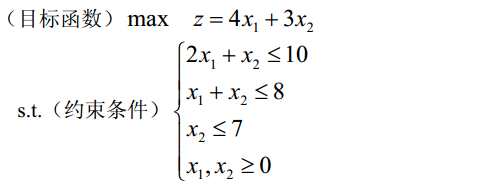而在matlab中使用matlab 标准的格式：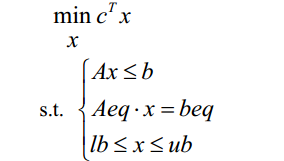若是目标函数是求解最大值的话，则取-C形式: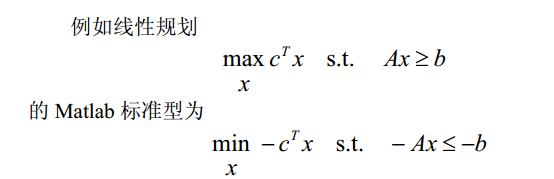具体的应用：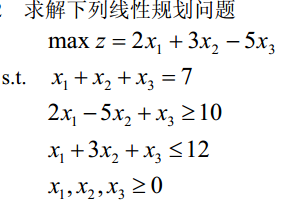代码：c = [2;3;-5];               %目标函数的系数
a = [-2,5,-1;1,3,1];        %不等式的系数（其中的不等式是小于等于）
b = [-10,12];               %不等式的右边的矩阵
aeq = [1,1,1];              %等式部分的系数
deq = 7;                    %等式的右边的值
x = linprog(-c,a,b,aeq,deq,zeros(3,1))
value = c'*x
结果：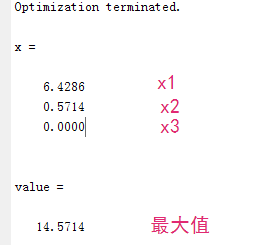（2）具体的函数linprog：使用help linproglinprog Linear programming.    X = linprog(f,A,b) attempts to solve the linear programming problem:              min f'*x    subject to:   A*x <= b              x     X = linprog(f,A,b,Aeq,beq) solves the problem above while additionally    satisfying the equality constraints Aeq*x = beq.     X = linprog(f,A,b,Aeq,beq,LB,UB) defines a set of lower and upper    bounds on the design variables, X, so that the solution is in    the range LB <= X <= UB. Use empty matrices for LB and UB    if no bounds exist. Set LB(i) = -Inf if X(i) is unbounded below;    set UB(i) = Inf if X(i) is unbounded above.     X = linprog(f,A,b,Aeq,beq,LB,UB,X0) sets the starting point to X0. This    option is only available with the active-set algorithm. The default    interior point algorithm will ignore any non-empty starting point.      X = linprog(PROBLEM) finds the minimum for PROBLEM. PROBLEM is a    structure with the vector 'f' in PROBLEM.f, the linear inequality    constraints in PROBLEM.Aineq and PROBLEM.bineq, the linear equality    constraints in PROBLEM.Aeq and PROBLEM.beq, the lower bounds in    PROBLEM.lb, the upper bounds in  PROBLEM.ub, the start point    in PROBLEM.x0, the options structure in PROBLEM.options, and solver    name 'linprog' in PROBLEM.solver. Use this syntax to solve at the    command line a problem exported from OPTIMTOOL. The structure PROBLEM    must have all the fields.     [X,FVAL] = linprog(f,A,b) returns the value of the objective function    at X: FVAL = f'*X.     [X,FVAL,EXITFLAG] = linprog(f,A,b) returns an EXITFLAG that describes    the exit condition of linprog. Possible values of EXITFLAG and the    corresponding exit conditions are       1  linprog converged to a solution X.      0  Maximum number of iterations reached.     -2  No feasible point found.     -3  Problem is unbounded.     -4  NaN value encountered during execution of algorithm.     -5  Both primal and dual problems are infeasible.     -7  Magnitude of search direction became too small; no further          progress can be made. The problem is ill-posed or badly          conditioned.     [X,FVAL,EXITFLAG,OUTPUT] = linprog(f,A,b) returns a structure OUTPUT    with the number of iterations taken in OUTPUT.iterations, maximum of    constraint violations in OUTPUT.constrviolation, the type of    algorithm used in OUTPUT.algorithm, the number of conjugate gradient    iterations in OUTPUT.cgiterations (= 0, included for backward    compatibility), and the exit message in OUTPUT.message.     [X,FVAL,EXITFLAG,OUTPUT,LAMBDA] = linprog(f,A,b) returns the set of    Lagrangian multipliers LAMBDA, at the solution: LAMBDA.ineqlin for the    linear inequalities A, LAMBDA.eqlin for the linear equalities Aeq,    LAMBDA.lower for LB, and LAMBDA.upper for UB.     NOTE: the interior-point (the default) algorithm of linprog uses a          primal-dual method. Both the primal problem and the dual problem          must be feasible for convergence. Infeasibility messages of          either the primal or dual, or both, are given as appropriate. The          primal problem in standard form is               min f'*x such that A*x = b, x >= 0.          The dual problem is               max b'*y such that A'*y + s = f, s >= 0. 
展开全文• 求解线性规划命令linprog使用格式： (1) x = linprog(C, A, b) [x,fval] = linprog(C, A, b) (2) x = linprog(C,A,b,Aeq,beq) [x,fval] = linprog(C,A,b,Aeq,beq) ...例子： C = [500,600]; A = [-1,-1;1,0;0,1;
求解线性规划命令linprog使用格式：
(1) x = linprog(C, A, b)
[x,fval] = linprog(C, A, b)
(2) x = linprog(C,A,b,Aeq,beq)
[x,fval] = linprog(C,A,b,Aeq,beq)
(3) x = linprog(C,A,b,Aeq,beq,Lb,Ub)
[x, fval] = linprog(C,A,b,Aeq,beq,Lb,Ub)
例子：
C = [500,600];
A = [-1,-1;1,0;0,1;]; %不等式：AX<b
b = [-5000;5000;3000];
Aeq = []; %等式：AeqX=beq
beq = [];
Lb = [0;0]; %变量范围：Lb<X<Ub
Ub = [inf;inf];
x = linprog(-C, A, b, Aeq, beq, Lb, Ub); %求最大值问题-C，求最小值问题C
z = C*x

结果：
x =  5000  3000
z =   4300000



展开全文• 一、首先将线性规划模型标准化为如下形式（注意要化成目标函数为min） ...三、例子 对应的matlab代码如下 f = [-0.05 -0.27 -0.19 -0.185 -0.185]; A = [0 0.025 0 0 0;0 0 0.015 0 0;0 0 0 0.055 0;0 0 0 0 0...
一、首先将线性规划模型标准化为如下形式（注意要化成目标函数为min）

f，x，b，beq，vlb，vub是向量，A和Aeq是矩阵

二、使用linprog求解（参数如下）

[x,fval]=linprog(f,A,b,Aeq,beq,vlb,vub)

三、例子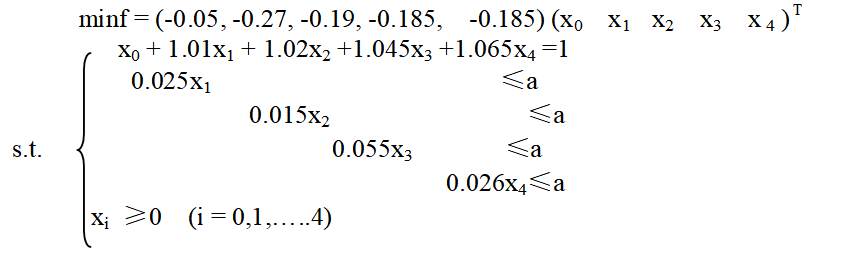对应的matlab代码如下

f = [-0.05 -0.27 -0.19 -0.185 -0.185]';
A = [0 0.025 0 0 0;0 0 0.015 0 0;0 0 0 0.055 0;0 0 0 0 0.026];
b = [a a a a]';
Aeq = [1 1.01 1.02 1.045 1.065];
beq = ;
vlb = [0 0 0 0 0]';
vub = [];
[x,val]=linprog(f,A,b,Aeq,beq,vlb,vub);


展开全文• 1)inprog函数是用来求解线性规划问题的。 什么是线性规划问题？...而在matlab中使用matlab 标准的格式： 若是目标函数是求解最大值的话，则取-C形式: 具体的应用： 代码： c = [2;3;-5]; %目标函数的系数 ...
• ## 使用 Matlab 解决数学建模问题

千次阅读 多人点赞 2018-12-06 00:35:37
线性规划函数1. 线性规划函数：linprog()1.1. linprog() 的标准形式1.2. linprog() 的使用1.3. 应用例子 1. 线性规划函数：linprog() ...Matlab 中规定线性规划的标准形式为 min⁡xcTxs.t.{Ax≤...
• 一、线性规划 什么是线性规划问题？ 线性规划是在一系列的线性条件的约束...在matlab中，linprog函数可以求解线性规划问题，用于寻找目标函数的最小值。 matlab中，规划模型的标注写法如下： （1）[x,fval]=linprog(f,线性规划
• 这里主要对于matlab优化工具箱进行整理 linprog求解线性规划： ...官方文档有例子，用到哪个根据目录检索，再官方文档查阅 linprog求解线性规划： 求解一个线性不等式、线性等式和边界的简单线性规划。 调用...
• Matlab学习语法一 1：zeros函数 例子： X = zeros(4) X = 4×4 0 0 0 0 0 0 0 0 0 0 0 0 0 0 0 0 2：drag函数 生成对角矩阵，如下例： A=[zeros(4,1),diag([0.025,0.015,0.055,0.026])]; 生成下图矩阵: 3...
• 一、Matlab线性规划标准型 min(c'*x) s.t.Ax <= B; 二、函数形式 linprog(c,a,b,aeq,beq,lb,ub,x0,options); %c为目标函数系数矩阵 %a为限制条件系数矩阵，b为（不）等号右边构成的矩阵 %aeq,beq为等号限制条件 %...
• 在利用matlab求线性规划的问题过程中，我们常用到的是linprog函数，下面我们将主要介绍一下linprog函数的常规用法。 例子 求解下列线性规划问题 可编写matlab程序为 c=[2;3;-5]; a=[-2,5,-1;1,3,1]; b=[-10;...数学建模
• 在《matlab解决有约束的二次规划问题》中，提到了线性规划问题（linear programming），而线性规划问题实际与二次规划问题十分相似，现在在matlab中实现求解 这是用于求解问题的linprog（）函数文档 同样使用一...
• 1、例子 2、MATLAB求解 2.1 代码 c=[2;3;1]; a=[1 4 2;3 2 0]; b=[8;6]; [x,y]=linprog(c,-a,-b,[],[],zeros(3,1)) 2.2 结果 Optimization terminated. x = 0.8066 1.7900 0.0166 y = 7.0000 3、l....
• 线性规划问题是最简单的有约束最优化问题，下面是一个例子： min -2x1-x2-4x3-3x4-x5 向量x s.t. 2x2+x3+4x4+2x5 3x1+4x2+5x3-x4-x5 ...matlab提供了 linprog函数来解线性规划问题，函数调用格式如下： 下
• 可先自己假设一个单投入单产出的例子，在纸上写出模型的规划式，然后对应linprog所需要的输入去编写，之后在拓展到多指标的情况(单指标只是多指标中的一种简单情形)。这两个模型代码搞明白后，再去比较非期望产出...
• 可先自己假设一个单投入单产出的例子，在纸上写出模型的规划式，然后对应linprog所需要的输入去编写，之后在拓展到多指标的情况(单指标只是多指标中的一种简单情形)。这两个模型代码搞明白后，再去比较非期望产出...
• 例子 程序文件 功能例6-1 xFminbnd.m 单变量函数的最小化例6-2 x1LinProg.m 求解线性规划问题例6-3 x2LinProg.m 求解线性规划问题例6-4 xFminsearch.m 用Nelder-Mead单纯形法求Rosen...
• [x,f_opt,flag,c]=linprog(f,A,B,Aeq,Beq,xm,xM,x0,opt) 参数说明： X: 解 f_opt: 最优值 Flag：大于零表示求解成功，否则求解出问题 C：求解信息 X0：搜索点的初值 Opt：最优化控制项 例子MATLAB...
• ##matlab中常用线性规划的代码和例子：## 1.代码：# 2.例子： 代码： f=[3;-2]; a=[2,3;2,1]; b=[18;10]; lb=[0,0]; [x,favl]=linprog(f,a,b,[],[],lb)%求出f(1)的极大值% clc,clear f=[-4;-3]; a=[2,3;2,1]; b=...git 算法
• matlab线性规划模型电子科技大学官方讲义，其中包括了基本理论，函数linprog的使用，以及一些实际例子
• 发现 scipy.optimize.linprog的算法支持: 单纯形法 和内点法, 在测试一个例子的时候, 运行不出来. 但在matlab中几个算法都可以. 单纯形法在matlab 已经不推荐使用. 现在用什么? GNU Linear Programming Kit CLP, ...
• 前言 线性规划可能看起来很简单，但其中也不乏比较精妙的处理方法。上了数模选修课之后才知道自己原来对建模的理解太浅，只知道想一种方法算出一个...附matlab源码（linprog） %M1.m clear;clc; original_data=[2.3...数学建模
• 2、Matlab 中规定线性规划的标准形式 min cx Ax<=b; Aeq.x=beq; lb<=x<=ub 3、基本函数形式 linprog(c,A,b)，它的返回值是向量 x 的值。 4、例子 4.1、解下列线性规划问题 c=[2,3,-5]; a=[-2,5,1;1,3.....算法
• 目 录 1 Matlab 软件 ........................................................................................................... 1 1.1 基本介绍.............................................................数学建模 编程
• 这几天在搞一个关于整数线性规划求解的程序，在实际例子测试时，总是出错，搞得现在头都:'( 晕沉沉的。希望高手们来帮忙看看，点拨一下:Q 。M文件：function [x,fval,status,output,bound]=fen(f,A,b,lb,ub,x0,...# linprog例子matlabmatlab 订阅